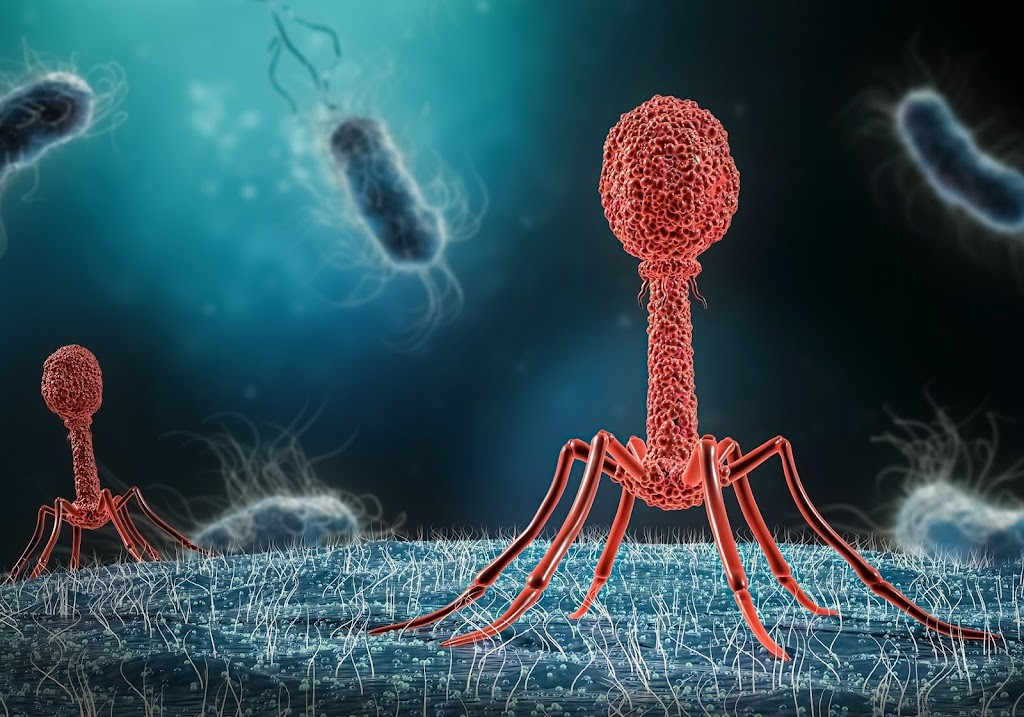8:02 pm

# The multiplicity of infection (MOI) of the phage explainedThe Multiplicity of Infection (MOI) is a crucial metric in bacteriophage research and is defined as the ratio of the number of infectious agents (e.g. phages or any other viruses) to the number of infection targets (e.g. bacterial cells). For example, if a culture of bacterial cells is inoculated with 100 phage particles, and the culture contains 10,000 bacterial cells, the MOI would be 100/10,000 = 0.01.

## Procedure to Obtain MOI

1. Isolate and purify the bacteriophage: This step is important to obtain a pure and concentrated sample of phages for accurate MOI calculation.
2. Count the number of infection targets: This can be done by counting the number of bacterial cells in the culture using a hemocytometer or other cell counting methods.
3. Inoculate the culture with a known number of phages: This can be done by adding a known volume of phage suspension to the culture.
4. Calculate MOI: Divide the number of phages inoculated by the number of bacterial cells in the culture (You can use our MOI calculator here).

## Interpretation

The MOI is a statistical measure and does not account for the variability in the number of phages that will enter each cell. Some cells may absorb multiple phages, while others may not absorb any. The probability of a cell absorbing n phages when inoculated with an MOI of m can be calculated using a Poisson distribution. The infectivity of the phages also affects this relationship.

For example, if an MOI of 1 (1 phage per cell) is used to infect a population of cells, the probability that a cell will not get infected is P(0) = 36.79%, and the probability that it is infected by a single phage is P(1) = 36.79%. If an MOI of 2 is used, the probability that a cell is infected by 2 phages is P(2) = 18.39%, and so on.

The average percentage of cells that will become infected as a result of inoculation with a given MOI can be obtained by realizing that it is simply P(n > 0) = 1 − P(0). Hence, the average fraction of cells that will become infected following inoculation with an MOI of m is given by:

It is important for bacteriophage researchers to understand the concept of MOI and how to calculate it. This information can be used to optimize phage infectivity experiments and to determine the most effective MOI for a specific phage-bacterial system.